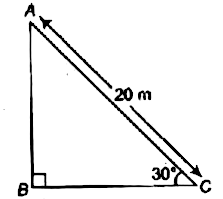Home/Class 10 Math Chapter List/11. Some Applications of Trigonometry/

# A circus artist is climbing a (20m) long rope, which is tightly stretched and tied from the top of a vertical pole to the ground. Find the height of the pole, if the angle made by the rope with the ground level is (30^{o}) (see figure).

## QuestionMathClass 10

A circus artist is climbing a $$20m$$ long rope, which is tightly stretched and tied from the top of a vertical pole to the ground. Find the height of the pole, if the angle made by the rope with the ground level is $$30^{o}$$ (see figure).$$10\ m$$
4.64.6## Solution

In the given figure, AB be the height of the pole and$$AC=20m$$ be the length of rope which is tied from the top of the pole.
We have to determine the height $$AB=?$$
$$\ln \Delta ABC,$$
$$\sin 30^{\circ }=\frac {AB}{AC}=\frac {AB}{20}$$
$$\Rightarrow$$ $$\frac {1}{2}=\frac {AB}{20}$$ [$$\because \sin30^{\circ}=\frac{1}{2}$$]
$$\Rightarrow$$ $$AB=\frac {20}{2}=10m$$
Hence, the height of the pole is $$10m$$